Next: Potential energy Up: Conservation of energy Previous: Work

## Conservative and non-conservative force-fields

Suppose that a non-uniform force-fieldacts upon an object which moves along a curved trajectory, labeled path 1, from pointto point. See Fig. 40. As we have seen, the workperformed by the force-field on the object can be written as a line-integral along this trajectory:(148)

Suppose that the same object moves along a different trajectory, labeled path 2, between the same two points. In this case, the workperformed by the force-field is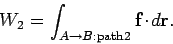(149)

Basically, there are two possibilities. Firstly, the line-integrals (148) and (149) might depend on the end points,and, but not on the path taken between them, in which case. Secondly, the line-integrals (148) and (149) might depend both on the end points,and, and the path taken between them, in which case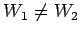(in general). The first possibility corresponds to what physicists term a conservative force-field, whereas the second possibility corresponds to a non-conservative force-field.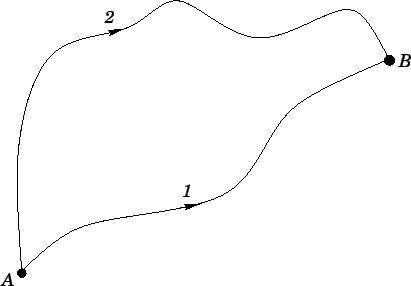What is the physical distinction between a conservative and a non-conservative force-field? Well, the easiest way of answering this question is to slightly modify the problem discussed above. Suppose, now, that the object moves from pointto pointalong path 1, and then from pointback to pointalong path 2. What is the total work done on the object by the force-field as it executes this closed circuit? Incidentally, one fact which should be clear from the definition of a line-integral is that if we simply reverse the path of a given integral then the value of that integral picks up a minus sign: in other words,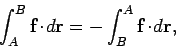(150)

where it is understood that both the above integrals are taken in opposite directions along the same path. Recall that conventional 1-dimensional integrals obey an analogous rule: i.e., if we swap the limits of integration then the integral picks up a minus sign. It follows that the total work done on the object as it executes the circuit is simply(151)

whereandare defined in Eqs. (148) and (149), respectively. There is a minus sign in front ofbecause we are moving from pointto point, instead of the other way around. For the case of a conservative field, we have. Hence, we conclude that(152)

In other words, the net work done by a conservative field on an object taken around a closed loop is zero. This is just another way of saying that a conservative field stores energy without loss: i.e., if an object gives up a certain amount of energy to a conservative field in traveling from pointto point, then the field returns this energy to the object--without loss--when it travels back to point. For the case of a non-conservative field,. Hence, we conclude that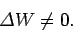(153)

In other words, the net work done by a non-conservative field on an object taken around a closed loop is non-zero. In practice, the net work is invariably negative. This is just another way of saying that a non-conservative field dissipates energy: i.e., if an object gives up a certain amount of energy to a non-conservative field in traveling from pointto point, then the field only returns part, or, perhaps, none, of this energy to the object when it travels back to point. The remainder is usually dissipated as heat.

What are typical examples of conservative and non-conservative fields? Well, a gravitational field is probably the most well-known example of a conservative field (see later). A typical example of a non-conservative field might consist of an object moving over a rough horizontal surface. Suppose, for the sake of simplicity, that the object executes a closed circuit on the surface which is made up entirely of straight-line segments, as shown in Fig. 41. Let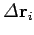represent the vector displacement of the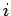th leg of this circuit. Suppose that the frictional force acting on the object as it executes this leg is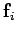. One thing that we know about a frictional force is that it is always directed in the opposite direction to the instantaneous direction of motion of the object upon which it acts. Hence,. It follows that. Thus, the net work performed by the frictional force on the object, as it executes the circuit, is given by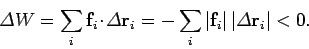(154)

The fact that the net work is negative indicates that the frictional force continually drains energy from the object as it moves over the surface. This energy is actually dissipated as heat (we all know that if we rub two rough surfaces together, sufficiently vigorously, then they will eventually heat up: this is how mankind first made fire) and is, therefore, lost to the system. (Generally speaking, the laws of thermodynamics forbid energy which has been converted into heat from being converted back to its original form.) Hence, friction is an example of a non-conservative force, because it dissipates energy rather than storing it.Next: Potential energy Up: Conservation of energy Previous: Work
Richard Fitzpatrick 2006-02-02